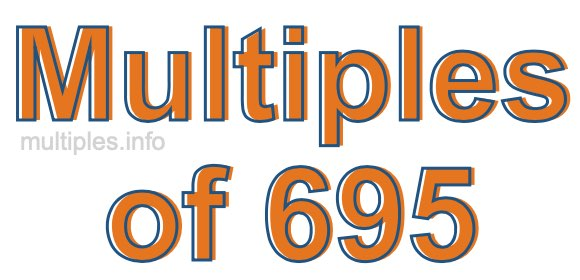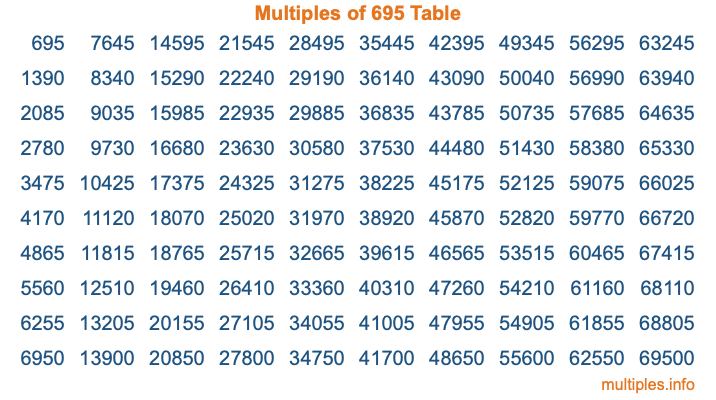Multiples of 695Welcome to the Multiples of 695 page. Here we will first teach you everything you will ever need to know about the multiples of 695, and then give you a study guide summary of everything we taught you to make sure you remember it all. Use this page to look up facts and learn information about the multiples of 695. This page will make you a multiples of six hundred ninety-five expert!

Definition of Multiples of 695
Multiples of 695 are all the numbers that when divided by 695 equal an integer. Each of the multiples of 695 are called a multiple. A multiple of 695 is created by multiplying 695 by an integer.

Therefore, to create a list of multiples of 695, you start with 1 multiplied by 695, then 2 multiplied by 695, then 3 multiplied by 695, and so on for as long as you want. Thus, the list of the first five multiples of 695 is 695, 1390, 2085, 2780, and 3475. To see a larger list of multiples of 695, see the printable image of Multiples of 695 further down on this page. We also have a category where you can choose any nth multiple of 695.

Multiples of 695 Checker
The Multiples of 695 Checker below checks to see if any number of your choice is a multiple of 695. In other words, it checks to see if there is any number (integer) that when multiplied by 695 will equal your number. To do that, we divide your number by 695. If the the quotient is an integer, then your number is a multiple of 695.

Is  a multiple of 695?

Least Common Multiple of 695 and ...
A Least Common Multiple (LCM) is the lowest multiple that two or more numbers have in common. This is also called the smallest common multiple or lowest common multiple and is useful to know when you are adding our subtracting fractions. Enter one or more numbers below (695 is already entered) to find the LCM.

Check out our LCM Calculator if you need more details about the Least Common Multiple or if you need the LCM for different numbers for adding and subtraction fractions.

nth Multiple of 695
As we stated above, 695 is the first multiple of 695, 1390 is the second multiple of 695, 2085 is the third multiple of 695, and so on. Enter a number below to find the nth multiple of 695.

th multiple of 695

Multiples of 695 vs Factors of 695
695 is a multiple of 695 and a factor of 695, but that is where the similarities end. All postive multiples of 695 are 695 or greater than 695. All positive factors of 695 are 695 or less than 695.

Below is the beginning list of multiples of 695 and the factors of 695 so you can compare:

Multiples of 695: 695, 1390, 2085, 2780, 3475, etc.

Factors of 695: 1, 5, 139, 695

As you can see, the multiples of 695 are all the numbers that you can divide by 695 to get a whole number. The factors of 695, on the other hand, are all the whole numbers that you can multiply by another whole number to get 695.

It's also interesting to note that if a number (x) is a factor of 695, then 695 will also be a multiple of that number (x).

Multiples of 695 vs Divisors of 695
The divisors of 695 are all the integers that 695 can be divided by evenly. Below is a list of the divisors of 695.

Divisors of 695: 1, 5, 139, 695

The interesting thing to note here is that if you take any multiple of 695 and divide it by a divisor of 695, you will see that the quotient is an integer.

Multiples of 695 Table
Below is an image of the first 100 multiples of 695 in a table. The table is in chronological order, column by column. The first column has the first ten multiples of 695, the second column has the next ten multiples of 695, and so on.The Multiples of 695 Table is also referred to as the 695 Times Table or Times Table of 695. You are welcome to print out our table for your studies.

Negative Multiples of 695
Although not often discussed or needed in math, it is worth mentioning that you can make a list of negative multiples of 695 by multiplying 695 by -1, then by -2, then by -3, and so on, to get the following list of negative multiples of 695:

-695, -1390, -2085, -2780, -3475, etc.

Multiples of 695 Summary
Below is a summary of important Multiples of 695 facts that we have discussed on this page. To retain the knowledge on this page, we recommend that you read through the summary and explain to yourself or a study partner why they hold true.

There are an infinite number of multiples of 695.

A multiple of 695 divided by 695 will equal a whole number.

695 divided by a factor of 695 equals a divisor of 695.

The nth multiple of 695 is n times 695.

The largest factor of 695 is equal to the first positive multiple of 695.

695 is a multiple of every factor of 695.

695 is a multiple of 695.

A multiple of 695 divided by a divisor of 695 equals an integer.

695 divided by a divisor of 695 equals a factor of 695.

Any integer times 695 will equal a multiple of 695.

Multiples of a Number
Here you can get the multiples of another number, all with the same attention to detail as we did for multiples of 695 on this page.

Multiples of
Multiples of 696
Did you find our page about multiples of six hundred ninety-five educational? Do you want more knowledge? Check out the multiples of the next number on our list!